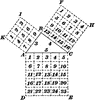# Right Triangle Showing Pythagorean TheoremIllustration of a right triangle that can be used to show Pythagorean theorem. “In any right triangle, the square described upon the hypotenuse is equal to the sum of the squares described upon the other two sides.”

### Keywords

triangles, pythagorean theorem, Right Triangle, triples

### Galleries

Miscellaneous Triangles

### Source

International Correspondence Schools I.C.S. Reference Library Mathematics (Scranton: International Textbook Company, 1905) Geometry & Trigonometry Pg. 11

TIFF (full resolution)

2301×2400, 362.5 KiB

Large GIF

981×1024, 67.7 KiB

Medium GIF

613×640, 38.1 KiB

Small GIF

306×320, 15.9 KiB